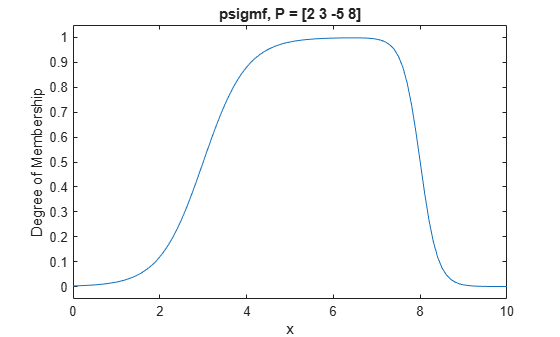# psigmf

Product of two sigmoidal membership functions

## Syntax

``y = psigmf(x,params)``

## Description

This function computes fuzzy membership values using the product of two sigmoidal membership functions. You can also compute this membership function using a `fismf` object. For more information, see fismf Object.

This membership function is related to the `sigmf` and `dsigmf` membership functions.

example

````y = psigmf(x,params)` returns fuzzy membership values computed using the product of two sigmoidal membership functions. Each sigmoidal function is given by:$\text{\hspace{0.17em}}\text{\hspace{0.17em}}\text{\hspace{0.17em}}\text{\hspace{0.17em}}\text{\hspace{0.17em}}f\left(x;{a}_{k},{c}_{k}\right)=\frac{1}{1+{e}^{-{a}_{k}\left(x-{c}_{k}\right)}}$To define the membership function parameters, specify `params` as the vector [a1 c1 a2 c2].Membership values are computed for each input value in `x`.```

## Examples

collapse all

Specify input values across the universe of discourse.

`x = 0:0.1:10;`

Evaluate membership function for the input values.

`y = psigmf(x,[2 3 -5 8]);`

Plot the membership function.

```plot(x,y) title('psigmf, P = [2 3 -5 8]') xlabel('x') ylabel('Degree of Membership') ylim([-0.05 1.05])```## Input Arguments

collapse all

Input values for which to compute membership values, specified as a scalar or vector.

Membership function parameters, specified as the vector [a1 c1 a2 c2]. Here, a1 and c1 are the parameters of the first sigmoidal function, and a2 and c2 are the parameters of the second sigmoidal function.

For each sigmoidal function, to open the function to the left or right, specify a negative or positive value for a, respectively. The magnitude of a defines the width of the transition area, and parameter c defines the center of the transition area.

To define a unimodal membership function with a maximum value of `1`, specify opposite signs for a1 and a2, and select c values far enough apart to allow for both transition areas to reach `1`.

## Output Arguments

collapse all

Membership value returned as a scalar or a vector. The dimensions of `y` match the dimensions of `x`. Each element of `y` is the membership value computed for the corresponding element of `x`.

## Alternative Functionality

### `fismf` Object

You can create and evaluate a `fismf` object that implements the `psigmf` membership function.

```mf = fismf("psigmf",P); Y = evalmf(mf,X);```

Here, `X`, `P`, and `Y` correspond to the `x`, `params`, and `y` arguments of `psigmf`, respectively.

## Version History

Introduced before R2006a